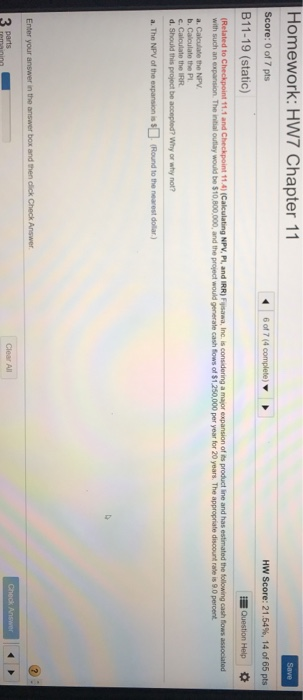### Create an Account

Home / Questions / (Related to Checkpoint 11.1 and Checkpoint 11.4) (Calculating N

# (Related to Checkpoint 11.1 and Checkpoint 11.4) (Calculating N

(Related to Checkpoint 11.1 and Checkpoint 11.4) (Calculating NPV, PI, and IRR) Fijisawa, Inc. is considering a major expansion of its product line and has estimated the following cash flows associated with such an expansion. The initial outlay would be \$10,800,000, and the project would generate cash flows of \$1,250,000 per year for 20 years. The appropriate discount rate is 9.0 percent.
a. Calculate the NPV.
b. Calculate the PI.
c. Calculate the IRR.
d. Should this project be accepted? Why or why not?Show transcribed image textSave Homework: HW7 Chapter 11 Score: 0 of 7 pts B11-19 (static) 6 of 7(4 complete) HW Score: 21.54%, 14 of 65 pts Question Help (Related to Checkpoint 11.1 and Checkpoint 114) Calculating NPV, PI, and IRR) Fywa, Inc. is considering a major expansion of product line and has estimated the following cash flows associated with such an expansion. The initial outlay would be \$10.800,000, and the project would generate cash flows of \$1,250,000 per year for 20 years. The appropriate discount rate is 9.0 percent a. Calculate the NPV b. Calculate the PL c. Calculate the RR d. Should this project be accepted? Why or why not? a. The NPV of the expansion is \$ (Round to the nearest dollar) Enter your answer in the answer box and then click Check Answer Check Answer 3 Parts Clear All

Aug 08 2020 View more View LessSubscribe To Get Solution